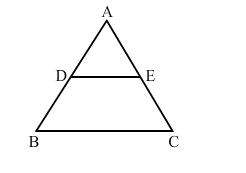# In an equilateral ∆ABC, D is the midpoint of AB and E is the midpoint of AC.

Question:

In an equilateral ∆ABCD is the midpoint of AB and E is the midpoint of AC. Then, ar(∆ABC) : ar(∆ADE) = ?(a) 2 : 1
(b) 4 : 1
(c) 1 : 2
(d) 1 : 4

Solution:

(b) 4:1
In ∆ABC, D is the midpoint of AB and E is the midpoint of AC.
Therefore, by midpoint theorem, DE">BC.
Also, by Basic Proportionality Theorem,

$\frac{A D}{D B}=\frac{A E}{E C}$

Also,

$A B=A C=B C(\because \Delta A B C$ is an equilateral triangle)

So, $\frac{A D}{D B}=\frac{A E}{E C}=1$

In $\triangle A B C$ and $\triangle A D E$, we have :

$\angle A=\angle A$

$\frac{A D}{A B}=\frac{A E}{A C}=\frac{1}{2}$

$\therefore \triangle A B C \sim \triangle A D E(S A S$ criterion)

$\therefore \operatorname{ar}(\triangle A B C): \operatorname{ar}(\triangle A D E)=(A B)^{2}:(A D)^{2}$

$\Rightarrow \operatorname{ar}(\triangle A B C): \operatorname{ar}(\triangle A D E)=2^{2}: 1^{2}$

$\Rightarrow a r(\triangle A B C): a r(\triangle A D E)=4: 1$